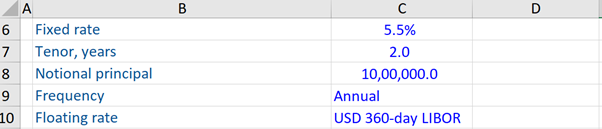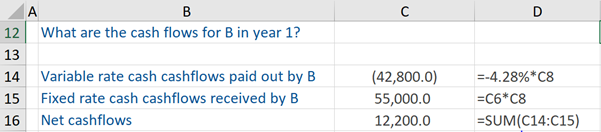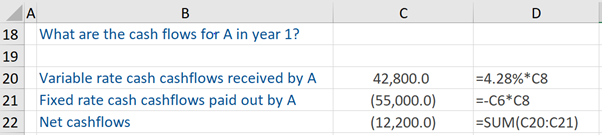## What are “Derivative Financial Instruments”?

A financial instrument derivative is a financial instrument whose value or performance is derived from or reliant on the fluctuations of the value of an underlying group of assets such as commodities, bonds, stocks, currencies, interest rates, and stock market indices. This financial instrument is itself usually a contract between two or more parties whose value is reliant on an underlying financial asset, such as those mentioned above.

Derivatives are an integral part of the investment environment. Multinational corporations, investment banks, and commercial banks are highly involved in derivatives trading. While derivatives could give exceptionally high returns, there is also a high risk associated with them.

## Key Learning Points

• Derivatives can be used to mitigate risk (such as in hedging) or to ‘take on’ risk (such as speculative investing)
• There are four basic types of derivatives – forward contracts, futures contracts, options contracts, and swaps
• One type of fixed income derivatives is called swaps – examples are interest rate, cross-currency, and equity swaps
• An example of an interest rate swap is where parties simply swap fixed interest payments for floating-rate payments

## Derivatives – Advantages, Risks, and Four Basic Types

Derivatives are used typically to hedge risks, frequently determined by the price of the underlying asset, provide access to markets or assets that would otherwise be unavailable, and enhance the efficiency of financial markets. However, there are risks associated with derivatives.

The same tends to be highly volatile, which exposes them to the probability of significant losses. Further, derivatives face the possibility of counterparty default. Moreover, they are often regarded as a tool of speculation, which can result in substantial losses.

There are four basic types of derivatives – Forward Contracts, Futures Contracts, Options Contracts, and Swaps. A forward contract is a customized contract between two parties to purchase or sell an asset at some specified price (decided in the present) at a future date.

A futures contract is very similar to a forward contract. However, unlike the latter, it is listed and traded on the exchange.

In an options contract, only one party is bound by the contract to purchase or sell an asset at some specified price at a future date. The other party is permitted to decide at a future date i.e at the expiration of the options contract. to exercise such an option, the other party pays a premium.

Swaps enable the parties involved to exchange their stream of cash flows. The most common one being swapping a fixed interest rate for a floating rate one.

## Interest Rate Swap

A common kind of fixed income derivative is interest rate swaps.

In an interest rate swap, the parties concerned simply swap fixed interest payments for floating-rate payments (or floating rate for floating rate). The floating interest is defined typically as LIBOR interest. Other types of interest rate swaps include cross-currency and equity swaps.

Given below is a workout of a fixed for floating interest rate swap, to gain insight into the basic cash flows of such a swap.

At the outset, the first party (A) enters into an arrangement with the second party (B) i.e. a fixed for floating interest rate swap arrangement with the second party. The date on which this interest rate swap was created, the USD 360 day LIBOR was 4.28%. The terms of this arrangement are stated below. With reference to the tenor which is 2 years, this effectively means that the 2-year swap rate when this trade was done was 5.5%.We are told the cash flows for party B in the first year. So, we calculate the variable rate cash flows that are paid out by them (i.e. -4.28% * US\$1,000,000 (notional principal)). Thereafter, since party B is the receiver, we calculate the fixed-rate cash flows received by them. This is calculated as 5.5% * US\$ 1,000,000 (notional principal).

The net cash flows for party B are equal to the difference between these two calculations.If we look at the opposite side of the trade – we are interested in the cash flows for party A, which is the payer (of the fixed-rate) in the first year. This party will receive variable or floating rate cash flows, which is calculated as 4.28% * US\$1,000,000 (notional principal). But this payer will pay the fixed-rate cash flows, which is calculated as -5.5% * US\$ 1,000,000 (notional principal).

The net cash flows for party A are equal to the difference between these two computations. To conclude, the cash flows from the first and the second party is a mirror image of each other.